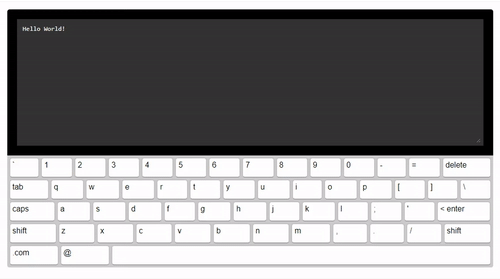## react-simple-keyboard

React Virtual Keyboard - Customizable, responsive and lightweight## Installation

`npm install react-simple-keyboard --save`

## Usage

``````import React, {Component} from 'react';
import Keyboard from 'react-simple-keyboard';
import 'simple-keyboard/build/css/index.css';

class App extends Component {

onChange = (input) => {
console.log("Input changed", input);
}

onKeyPress = (button) => {
console.log("Button pressed", button);
}

render(){
return (
<Keyboard
onChange={input =>
this.onChange(input)}
onKeyPress={button =>
this.onKeyPress(button)}
/>
);
}
}

export default App;
``````

## Options

You can customize the Keyboard by passing options (props) to it.
Here are the available options (the code examples are the defaults):

### layout

Modify the keyboard layout

``````layout={{
'default': [
'` 1 2 3 4 5 6 7 8 9 0 - = {bksp}',
'{tab} q w e r t y u i o p [ ] \\',
'{lock} a s d f g h j k l ; \' {enter}',
'{shift} z x c v b n m , . / {shift}',
'.com @ {space}'
],
'shift': [
'~ ! @ # \$ % ^ & * ( ) _ + {bksp}',
'{tab} Q W E R T Y U I O P { } |',
'{lock} A S D F G H J K L : " {enter}',
'{shift} Z X C V B N M < > ? {shift}',
'.com @ {space}'
]
}}
``````

### layoutName

Specifies which layout should be used.

``````layoutName={"default"}
``````

### display

Replaces variable buttons (such as `{bksp}`) with a human-friendly name (e.g.: "delete").

``````display={{
'{bksp}': 'delete',
'{enter}': '< enter',
'{shift}': 'shift',
'{s}': 'shift',
'{tab}': 'tab',
'{lock}': 'caps',
'{accept}': 'Submit',
'{space}': ' ',
'{//}': ' '
}}
``````

### theme

A prop to add your own css classes. You can add multiple classes separated by a space.

``````theme={"hg-theme-default"}
``````

### debug

Runs a console.log every time a key is pressed. Displays the buttons pressed and the current input.

``````debug={false}
``````

### newLineOnEnter

Specifies whether clicking the "ENTER" button will input a newline (`\n`) or not.

``````newLineOnEnter={false}
``````

### inputName

Allows you to use a single simple-keyboard instance for several inputs.

``````inputName={"default"}
``````

### onKeyPress

Executes the callback function on key press. Returns button layout name (i.e.: "{shift}").

``````onKeyPress={(button) => console.log(button)}
``````

### onChange

Executes the callback function on input change. Returns the current input's string.

``````onChange={(input) => console.log(input)}
``````

### onChangeAll

Executes the callback function on input change. Returns the input object with all defined inputs. This is useful if you're handling several inputs with simple-keyboard, as specified in the "Using several inputs" guide.

``````onChangeAll={(inputs) => console.log(inputs)}
``````

## Methods

simple-keyboard has a few methods you can use to further control it's behavior.
To access these functions, you need a `ref` of the simple-keyboard component, like so:

``````<Keyboard
ref={r => this.keyboard = r}
[...]
/>

// Then, on componentDidMount ..
this.keyboard.methodName(params);
``````

### clearInput

Clear the keyboard's input.

``````// For default input (i.e. if you have only one)
this.keyboard.clearInput();

// For specific input
// Must have been previously set using the "inputName" prop.
this.keyboard.clearInput("inputName");
``````

### getInput

Get the keyboard's input (You can also get it from the onChange prop).

``````// For default input (i.e. if you have only one)
let input = this.keyboard.getInput();

// For specific input
// Must have been previously set using the "inputName" prop.
let input = this.keyboard.getInput("inputName");
``````

### setInput

Set the keyboard's input. Useful if you want the keybord to initialize with a default value, for example.

``````// For default input (i.e. if you have only one)
this.keyboard.setInput("Hello World!");

// For specific input
// Must have been previously set using the "inputName" prop.
this.keyboard.setInput("Hello World!", "inputName");
``````

It returns a promise, so if you want to run something after it's applied, call it as so:

``````let inputSetPromise = this.keyboard.setInput("Hello World!");

inputSetPromise.then((result) => {
console.log("Input set");
});
``````

## Use-cases

### Using several inputs

Set the inputName option for each input you want to handle with simple-keyboard.

For example:

``````// Tell simple-keyboard which input is active
setActiveInput = (event) => {
this.setState({
inputName: event.target.id
});
}

// When the inputs are changed
// (retrieves all inputs as an object instead of just the current input's string)
onChangeAll = (input) => {
this.setState({
input: input
}, () => {
console.log("Inputs changed", input);
});
}

render(){
return (
<div>
<input id="input1" onFocus={this.setActiveInput} value={this.state.input['input1'] || ""}/>
<input id="input2" onFocus={this.setActiveInput} value={this.state.input['input2'] || ""}/>

<Keyboard
ref={r => this.keyboard = r}
inputName={this.state.inputName}
onChangeAll={inputs => this.onChangeAll(inputs)}
layoutName={this.state.layoutName}
/>
</div>
);
``````